##### The Japanese Bonsai specialist
Direct order Contact Help / Services Newsletter# Bassin granite 50 cm shinobutsu shozubachi

Tsukubai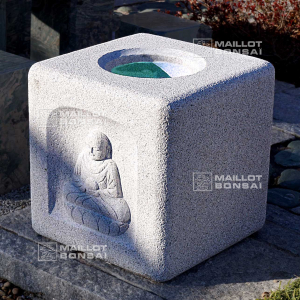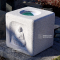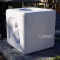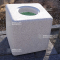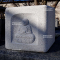ref. : 11580

630,00

voluminous/heavy item extra shipping of 45,00

Available quantity : 1Order

###### Description

Granite tsukubai basin with a pattern on a face. Dimensions : 50*50*50 cm on the outside. Height of the central hole : 10 cm. Diameter of the inside : 26 cm. Weight : 230 kilograms.

This model has a central hole that retains water. It is one of the elements composing the tsukubai. It is named shozubachi. This rock often goes with a bamboo dispenser nozzle discharging water that overflows in a simple closed circuit system.

Our garden items (basins, granite lanterns, rocks and japanese cobblestones) are stored outside. Indeed, the patina and the old aspect obtained (lichen and moss) give an additional authenticity charm, usually really appreciated in the traditional japanese garden art.

Here is a selection of pump models on the Conrad french website :

Think to see the backflow height.

This model, with its traditional aspect is a crush.

#granite 4.7 #conrad 4.6 #water 4.5 #shozubachi 4 #https 3.5 #basin 3.5 #this 3.4 #pump 3.4 #with 3.4 #traditional 3.1

Formule
(( ROUND((CHAR_LENGTH(b.article_nom)-CHAR_LENGTH(REPLACE(b.article_nom, 'granite', '')))/LENGTH('granite')) + ROUND((CHAR_LENGTH(b.article_description)-CHAR_LENGTH(REPLACE(b.article_description, 'granite', '')))/LENGTH('granite')) ) * 4.7) + (( ROUND((CHAR_LENGTH(b.article_nom)-CHAR_LENGTH(REPLACE(b.article_nom, 'conrad', '')))/LENGTH('conrad')) + ROUND((CHAR_LENGTH(b.article_description)-CHAR_LENGTH(REPLACE(b.article_description, 'conrad', '')))/LENGTH('conrad')) ) * 4.6) + (( ROUND((CHAR_LENGTH(b.article_nom)-CHAR_LENGTH(REPLACE(b.article_nom, 'shozubachi', '')))/LENGTH('shozubachi')) + ROUND((CHAR_LENGTH(b.article_description)-CHAR_LENGTH(REPLACE(b.article_description, 'shozubachi', '')))/LENGTH('shozubachi')) ) * 4) + (( ROUND((CHAR_LENGTH(b.article_nom)-CHAR_LENGTH(REPLACE(b.article_nom, 'https', '')))/LENGTH('https')) + ROUND((CHAR_LENGTH(b.article_description)-CHAR_LENGTH(REPLACE(b.article_description, 'https', '')))/LENGTH('https')) ) * 3.5) + (( ROUND((CHAR_LENGTH(b.article_nom)-CHAR_LENGTH(REPLACE(b.article_nom, 'this', '')))/LENGTH('this')) + ROUND((CHAR_LENGTH(b.article_description)-CHAR_LENGTH(REPLACE(b.article_description, 'this', '')))/LENGTH('this')) ) * 3.4) + (( ROUND((CHAR_LENGTH(b.article_nom)-CHAR_LENGTH(REPLACE(b.article_nom, 'pump', '')))/LENGTH('pump')) + ROUND((CHAR_LENGTH(b.article_description)-CHAR_LENGTH(REPLACE(b.article_description, 'pump', '')))/LENGTH('pump')) ) * 3.4) + (( ROUND((CHAR_LENGTH(b.article_nom)-CHAR_LENGTH(REPLACE(b.article_nom, 'with', '')))/LENGTH('with')) + ROUND((CHAR_LENGTH(b.article_description)-CHAR_LENGTH(REPLACE(b.article_description, 'with', '')))/LENGTH('with')) ) * 3.4) + (( ROUND((CHAR_LENGTH(b.article_nom)-CHAR_LENGTH(REPLACE(b.article_nom, 'traditional', '')))/LENGTH('traditional')) + ROUND((CHAR_LENGTH(b.article_description)-CHAR_LENGTH(REPLACE(b.article_description, 'traditional', '')))/LENGTH('traditional')) ) * 3.1) + (( ROUND((CHAR_LENGTH(b.article_nom)-CHAR_LENGTH(REPLACE(b.article_nom, 'shinobutsu', '')))/LENGTH('shinobutsu')) + ROUND((CHAR_LENGTH(b.article_description)-CHAR_LENGTH(REPLACE(b.article_description, 'shinobutsu', '')))/LENGTH('shinobutsu')) ) * 3) + (( ROUND((CHAR_LENGTH(b.article_nom)-CHAR_LENGTH(REPLACE(b.article_nom, 'japanese', '')))/LENGTH('japanese')) + ROUND((CHAR_LENGTH(b.article_description)-CHAR_LENGTH(REPLACE(b.article_description, 'japanese', '')))/LENGTH('japanese')) ) * 2.8)

## Secure payment## Delivery

Our logistic partners :04 74 55 23 48
Pépinière MAILLOT-BONSAÏ
Le Bois Frazy
01990 RELEVANT - FRANCE
on appointment